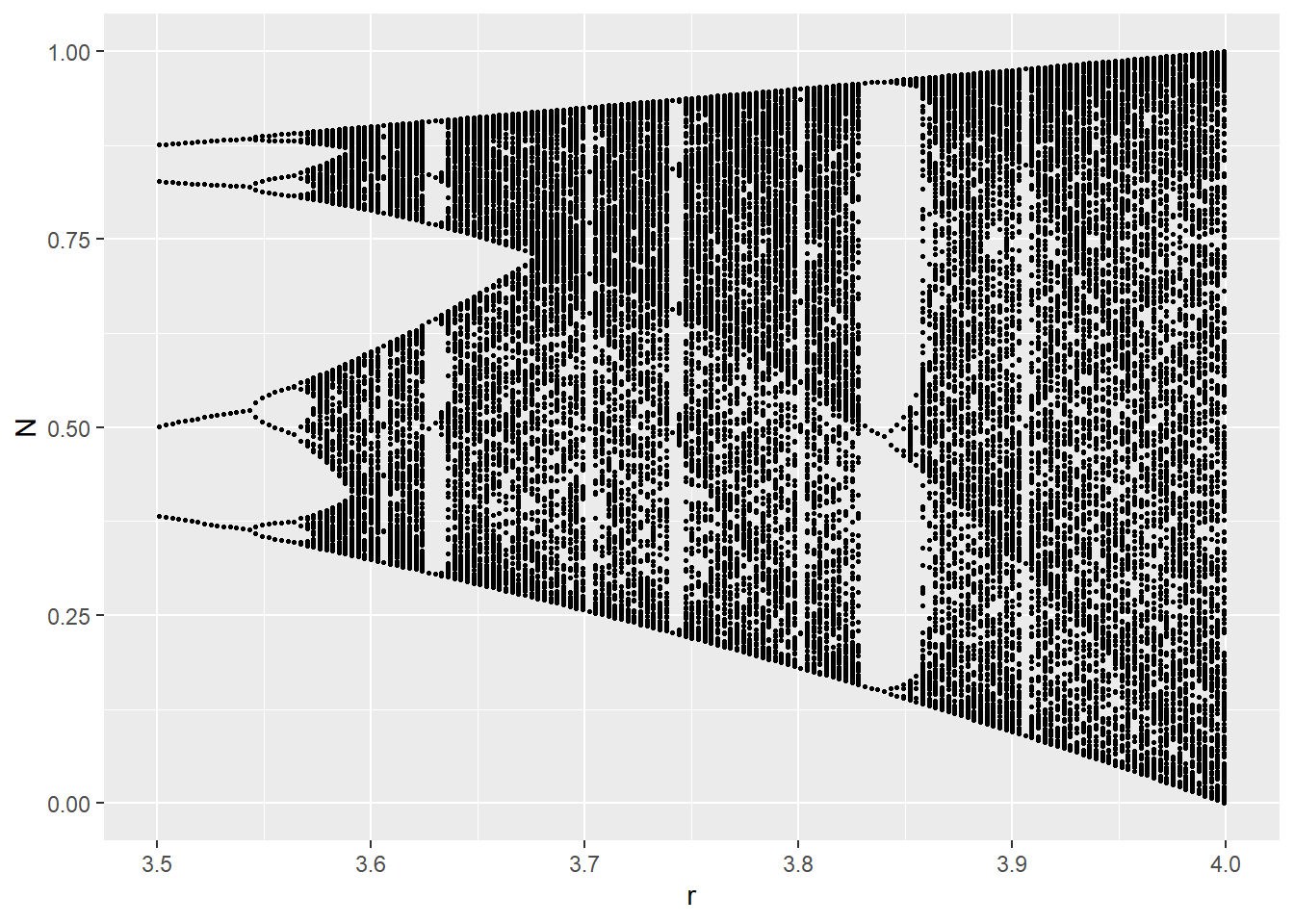# Objective

If you’ve ever wondered how logistic population growth (the Verhulst model), S curves, the logistic map, bifurcation diagrams, sensitive dependence on initial conditions, “orbits”, deterministic chaos, and Lyapunov exponents are related to one another… this post explains it in just 10 steps, each with some code in R so you can explore it all yourself. I’ve included some code written by other people who have explored this problem (cited below) as portions of my own code.

It all starts with a hypothesized population… and a process where the size of the population changes over time. We want to understand how (and under what conditions) those changes occur, so we choose a model that characterizes population changes: the logistic growth model. It’s been used in biology, ecology, econometrics, marketing, and other areas.

## Step 1

The logistic growth model describes how the size of a population (N) changes over time (t), based on some maximum population growth rate (r). There is a limiting factor called the carrying capacity (K) which represents the total population that the environment could support, based on the amount of available resources. dN/dt is the rate of change of the population over time.

$\frac{dN}{dt} = \frac{rN(K\:-\:N)}{K}$

## Step 2

You can simplify the logistic growth model by defining a new variable x to represent the portion of the population that’s alive, compared to the total population that the environment could support (and keep alive). So with x = N/K, you get a new differential equation in terms of x. Now we are looking at the rate of change of the “population fraction” over time. Once the population reaches its carrying capacity at x = N/K = 1, the environment can’t support any more members in the population:

$\frac{dx}{dt} = rx(1\:-\:x)$

## Step 3

You can solve this equation by integration! Then, you’ll have an expression that you can use to calculate x (which is still the population fraction) for any time t. The resulting equation is called the sigmoid or (more commonly), the S Curve. To compute x at any time t, all we need to know is how big the population was when we started looking at it (x0) and the maximum growth rate r:

$x(t) = \frac{1} {1\:+\:\big( \frac{1}{x_{0}} -1\big) + e^{-rt}}$

## Step 4

The equation for the S Curve is deterministic and continuous. If we want to solve it numerically, we have to discretize it by chopping up that continuous axis that contains time into little tiny pieces of time. That’s what produces the difference equation that we recognize as the logistic map below. It’s a map because it “maps” each value of the sequence onto the next value in the sequence.

As long as you know one of those values for x (indicated by the subscript n), you’ll be able to figure out the next value of x (indicated by the subscript n+1). The value x[n] is the population fraction of the current generation, and the value x[n+1] is the population fraction for the next generation. This makes the logistic map a Markov chain. If you plot x[n] on the x axis and x[n+1] on the y axis, this expression will produce the familiar upside down parabola:

$x_{n+1} = rx_{n}\: (1-x_{n})$

## Step 5

The logistic map behaves differently depending upon the maximum growth rate (r) that describes your population. This parameter is also called fecundity and represents how rabbit-like your population is reproducing. The higher the r, the more productive, like rabbits (although I’m not sure precisely which r you’d choose if you were studying rabbits). Here is an R function that you can use to generate the last M iterations from a sequence of N total:

logistic.map <- function(r, x, N, M) {
## from http://www.magesblog.com/2012/03/logistic-map-feigenbaum-diagram.html
## r: bifurcation parameter
## x: initial value, something greater than 0 and less than 1
## N: number of iterations (total)
## M: number of iteration points to be returned
z <- 1:N
z <- x
for(i in c(1:(N-1))){
z[i+1] <- r *z[i] * (1 - z[i])
}
## Return the last M iterations
z[c((N-M):N)]
}

## Step 6

The logistic map has many interesting properties, but here are two in particular (the first in Step 6 and the second in Step 7). First, for several values you can choose for r, the chain converges to a single value (or fixed point) when n gets really big. For other values of r, the value of x will eventually bounce between two values instead of converging (a limit cycle of 2). For other values of r, the value of x will eventually bounce between four values instead of converging. Sometimes, x will bounce around a near limitless collection of values (a condition called deterministic chaos). The eventual values (or collection of eventual values, if they bounce between values) is called an orbit. For example, when the growth rate r is 2.6, the logistic map rapidly converges to an orbit of about 0.615:

library(tidyverse)

iter <- logistic.map(2.6,.01,20,20)
ggplot(data.frame(index=1:length(iter), logistic.map=iter), aes(x=index,y=logistic.map)) + geom_line()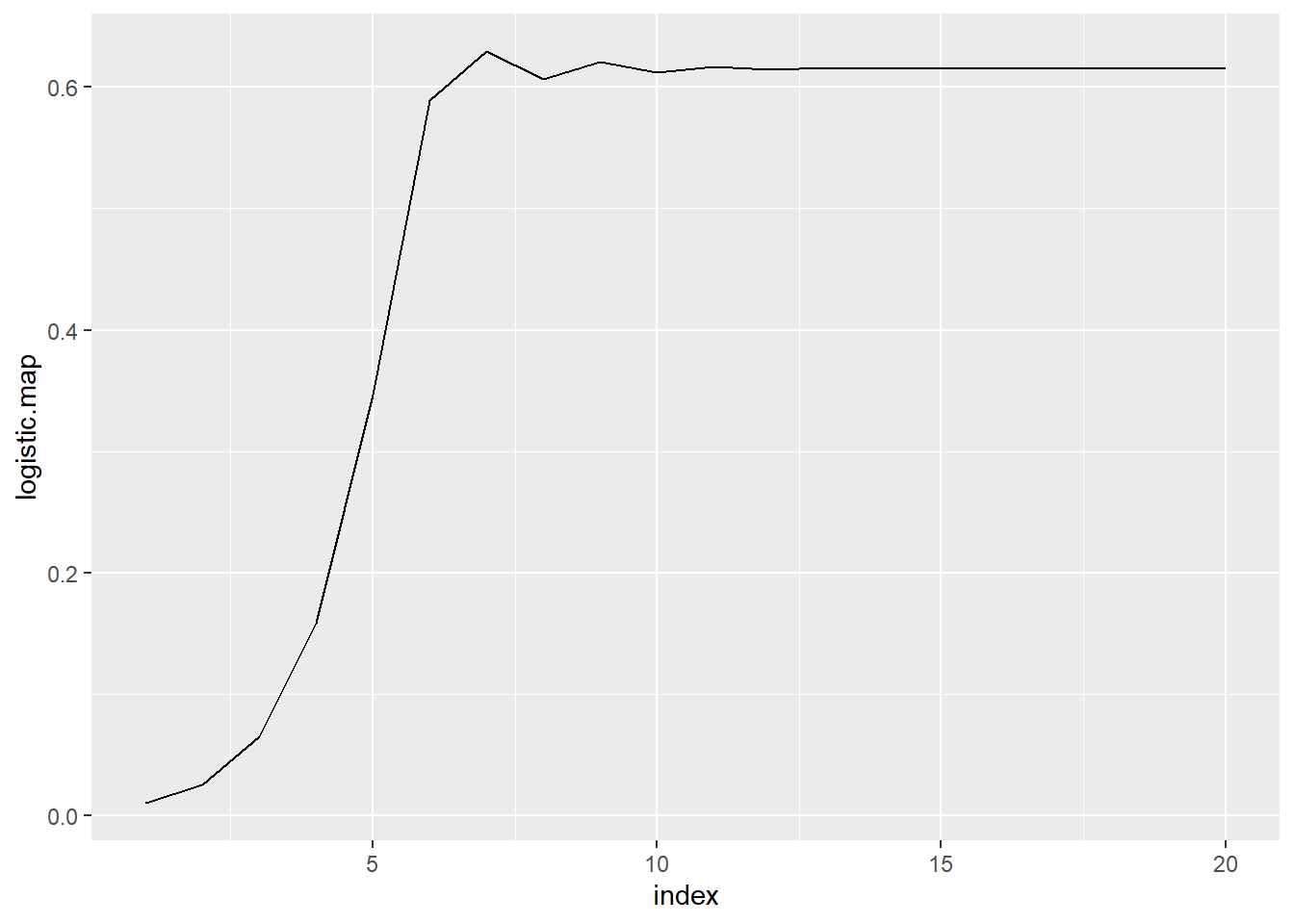## Step 7

Sometimes, it can be nice to take a look at how the values bounce around, and where they eventually converge (or not). To do this, we use cobweb diagrams (which are also sometimes called web diagrams). I used a function that I found at http://bayesianbiologist.com to plot the behavior of the orbits for r=2.6, r=3.2, and r=3.9:

set.seed(1234)

logistic.cobweb <- function(r, N=100) {
x<- seq(0,1,length=100)
x_next <- lapply(2:N, function(i) r*x[i]*(1-x[i]))
orbit.df <- as_tibble(cbind(x[2:N], unlist(x_next)))

p <- orbit.df %>% ggplot() + geom_line(aes(x=V1, y=V2, col="red"), size=2) +
xlab(expression(x[t])) + ylab(expression(x[t+1])) + ggtitle(paste0("r = ",r)) +
theme(axis.title.x=element_text(colour='black', size=18)) +
theme(axis.title.y=element_text(colour='black', size=18)) +
theme(legend.position="none")

start=runif(1,0,1)

vert=FALSE
seg_df <- data.frame(x=start, xend=start, y=0, yend=r*start*(1-start))
for(i in 1:(2*N)) {
if(vert) {
seg_df <- rbind(seg_df, data.frame(x=start, xend=start, y=start, yend=r*start*(1-start)))
vert=FALSE
} else {
seg_df <- rbind(seg_df, data.frame(x=start, xend=r*start*(1-start), y=r*start*(1-start), yend=r*start*(1-start)))
vert=TRUE
start=r*start*(1-start)
}
}
p + geom_segment(data=seg_df, aes(x=x, xend=xend, y=y, yend=yend))
}

library(cowplot)

g0 <- logistic.cobweb(2)
g1 <- logistic.cobweb(2.6)
g2 <- logistic.cobweb(3.3)
g3 <- logistic.cobweb(3.9)

cowplot::plot_grid(g0, g1, g2, g3)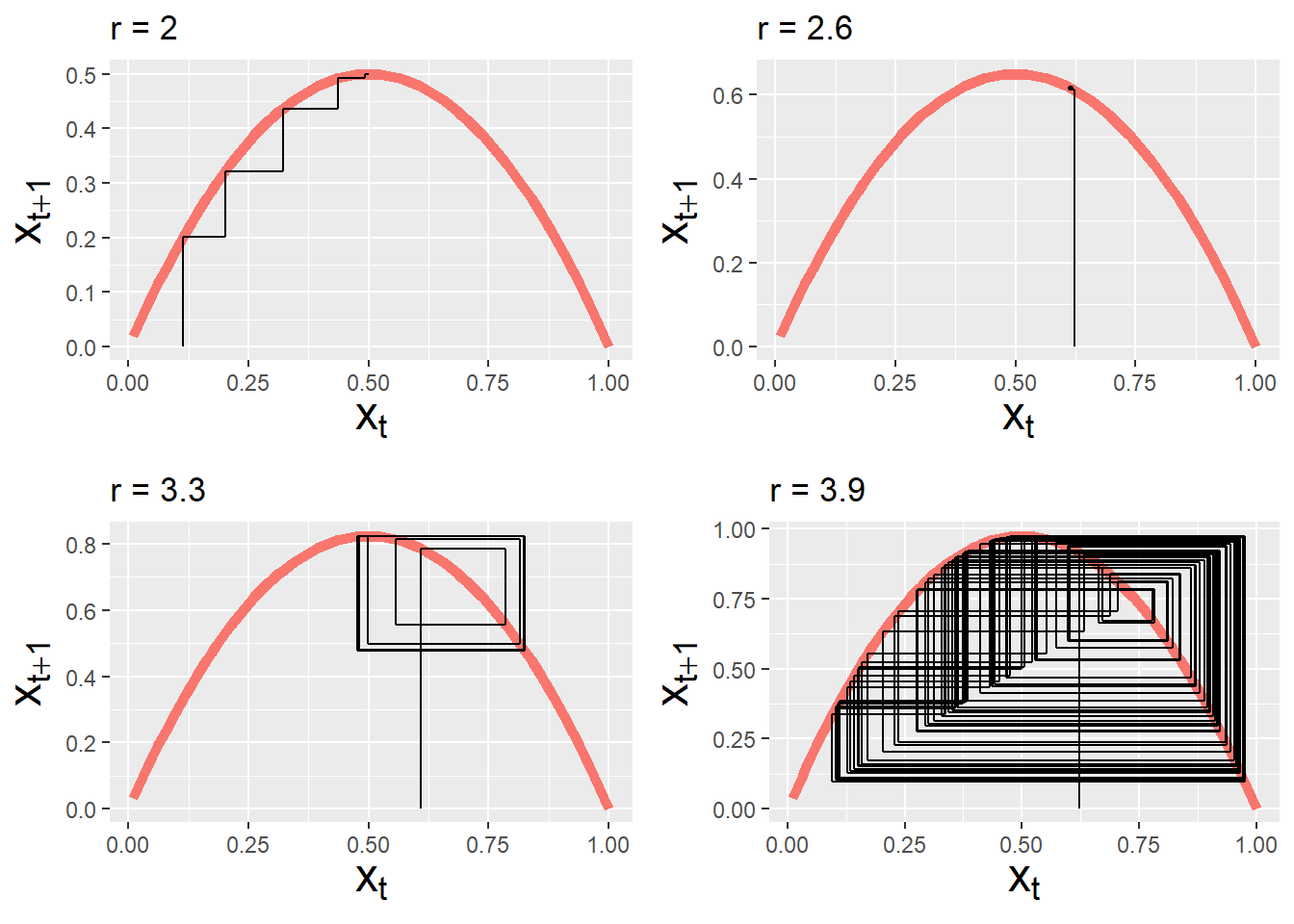## Step 8

Second, for some values of r, the logistic map shows sensitive dependence on initial conditions. For example, let’s see what happens for two different growth rates (r=3 and r=3.9) when we start one iteration with an x[n] of 0.5 COLORED BLACK, and another one with an x[n] of 0.5001 COLORED RED. It’s a small, small difference that can lead to big, BIG variations in the orbits. In the r=3 case, the chain produced by the logistic map with x[n] of 0.5 (in black) is IDENTICAL to the chain produced by the logistic map with x[n] of 0.5001 (in red). That’s why you can’t see the black… the values are the same!

first <- logistic.map(3,.5,120,100)
second <- logistic.map(3,.5001,120,100)
ggplot() + geom_line(data=data.frame(index=1:length(first), logistic.map=first), aes(x=index,y=logistic.map, colour="red")) +
geom_line(data=data.frame(index=1:length(second), logistic.map=second), aes(x=index,y=logistic.map))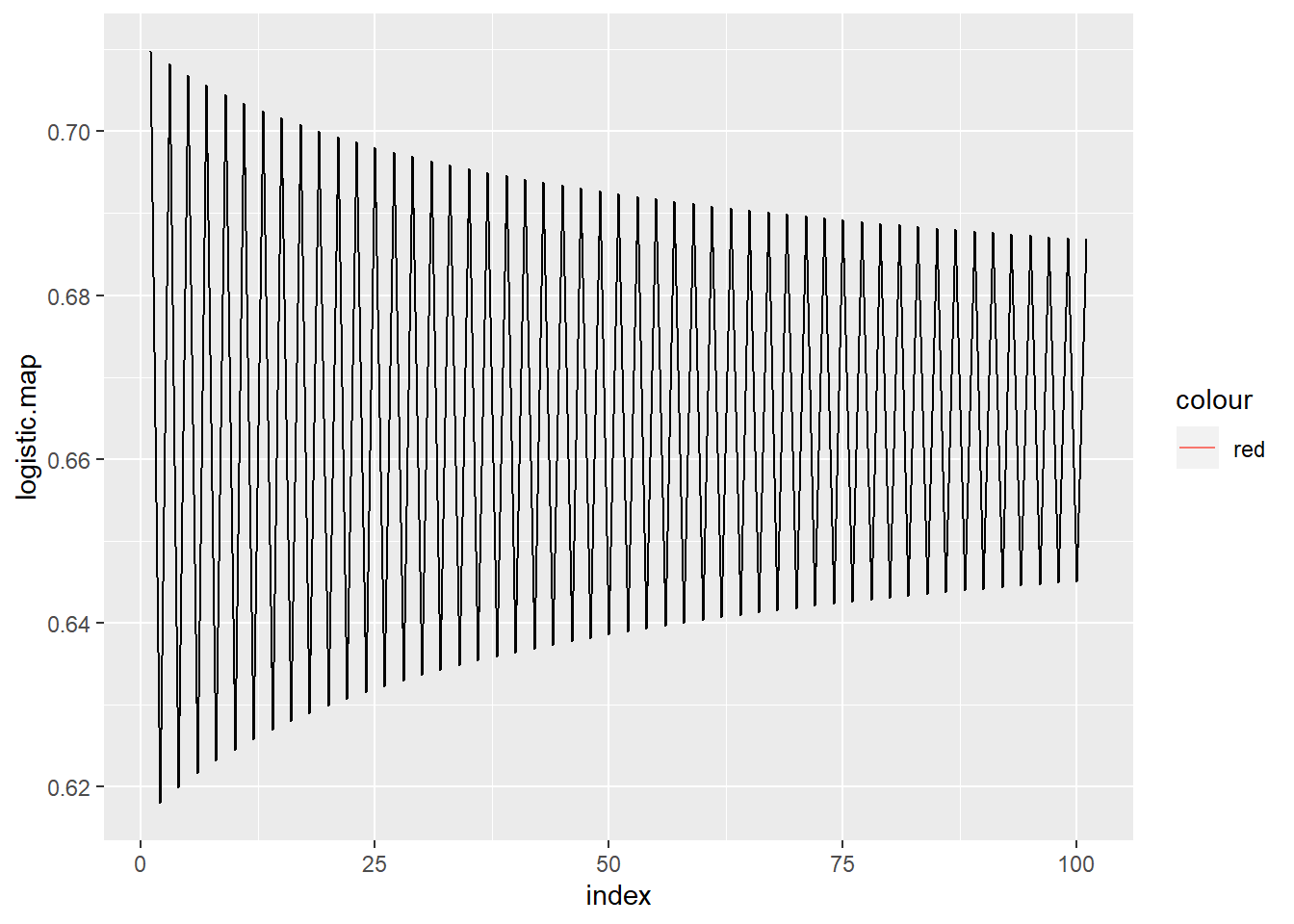But for the r=3.9 case, the chain produced by the logistic map with x[n] of 0.5 (in black) RAPIDLY DIVERGES from the chain produced by the logistic map with x[n] of 0.5001 (in red). They are very different, despite a very tiny difference in initial conditions! The logistic map for r=3.9 shows a very sensitive dependence on initial conditions.

first <- logistic.map(3.9,.5,120,100)
second <- logistic.map(3.9,.5001,120,100)
ggplot() + geom_line(data=data.frame(index=1:length(first), logistic.map=first), aes(x=index,y=logistic.map,colour="red")) +
geom_line(data=data.frame(index=1:length(second), logistic.map=second), aes(x=index,y=logistic.map))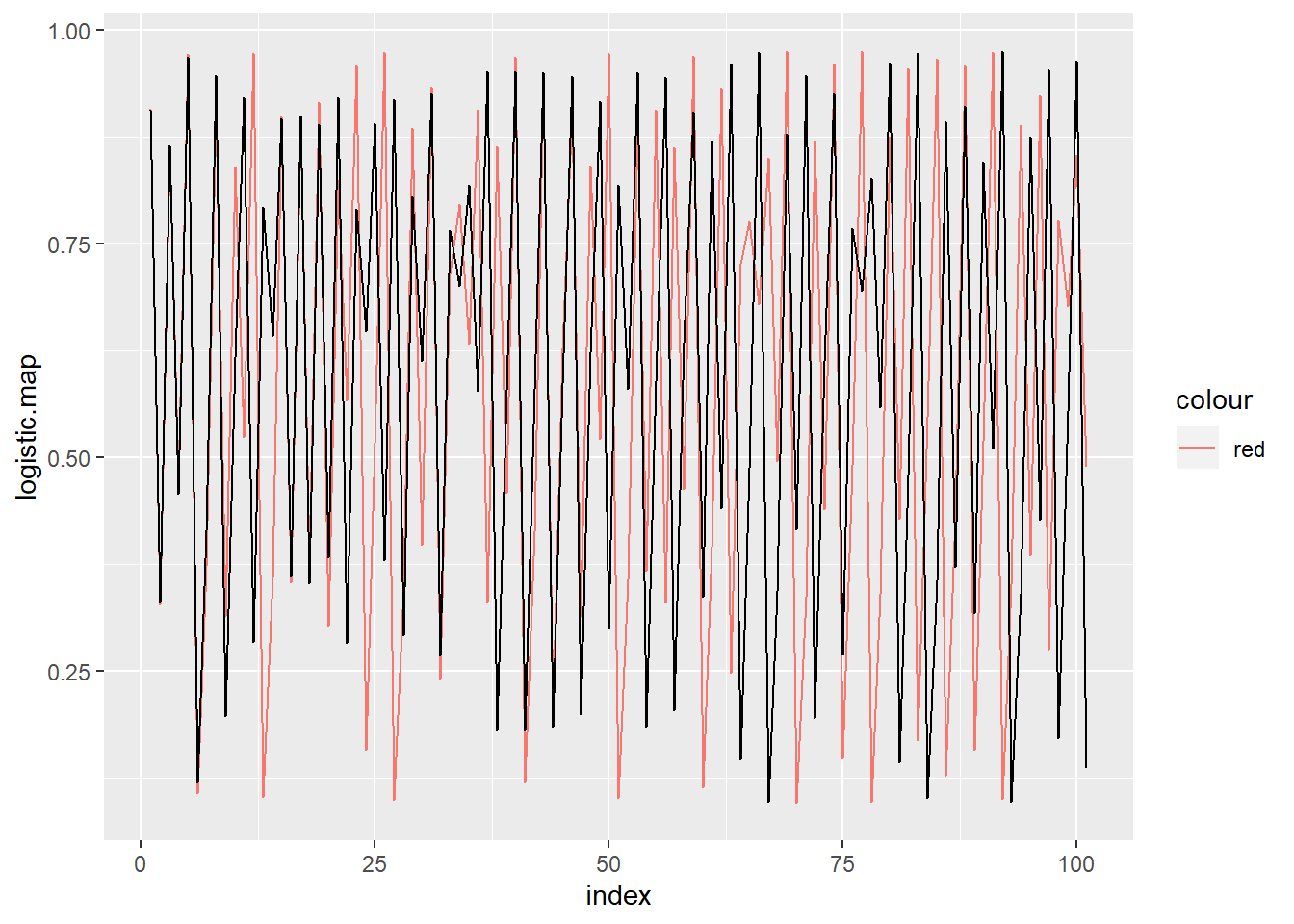## Step 9

For any chain, we can determine just how sensitive the logistic map is to initial conditions by looking at the Lyapunov exponent. Very simplistically, if the Lyapunov exponent is negative, the chain will converge to one or more fixed points for that value of r. If the Lyapunov exponent is positive, the chain will demonstrate deterministic chaos for that value of r. If the Lyapunov exponent is zero, there is a bifurcation: a 1-cycle is doubling to a 2-cycle, a 2-cycle is doubling to a 4-cycle, or so forth. The top chart shows an approximation of the Lyapunov exponent based on the first 500 iterations (ideally, you’d use an infinite number, but that would eat up too much computing time), and the bottom chart shows a bifurcation diagram. You’ll notice that the Lyapunov exponents are zero where a bifurcation occurs. To interpret the bifurcation diagram, just remember that each vertical slice through it represents the results of ONE COMPLETELY CONVERGED CHAIN from the logistic map. So it shows the results from many, many, many completely converged chains – and provides an excellent way for us to look at the behavior of MANY different types of populations in just one chart:

x <- seq(0,4,0.01)
XI <- lya <- 0

# Create 400 different logistic maps for r=0.00 to 4.00 and get one Lyapunov exponent (lya) from each
for (i in 1:400) {
xi <- logistic.map(x[i],.01,500,500)
XI <- rbind(XI,xi)
lya[i] <- sum(log(abs(x[i]-(2*x[i]*XI[i,]))))/length(x)
}

ggplot() + geom_line(data=data.frame(index=1:length(lya), lya=lya), aes(x=index,y=lya), size=1.2) +
geom_hline(yintercept=0, col="red") + geom_vline(xintercept=300, col="blue") +
scale_y_continuous(limits = c(-5,1)) + ggtitle("Lyapunov Exponents for Logistic Map")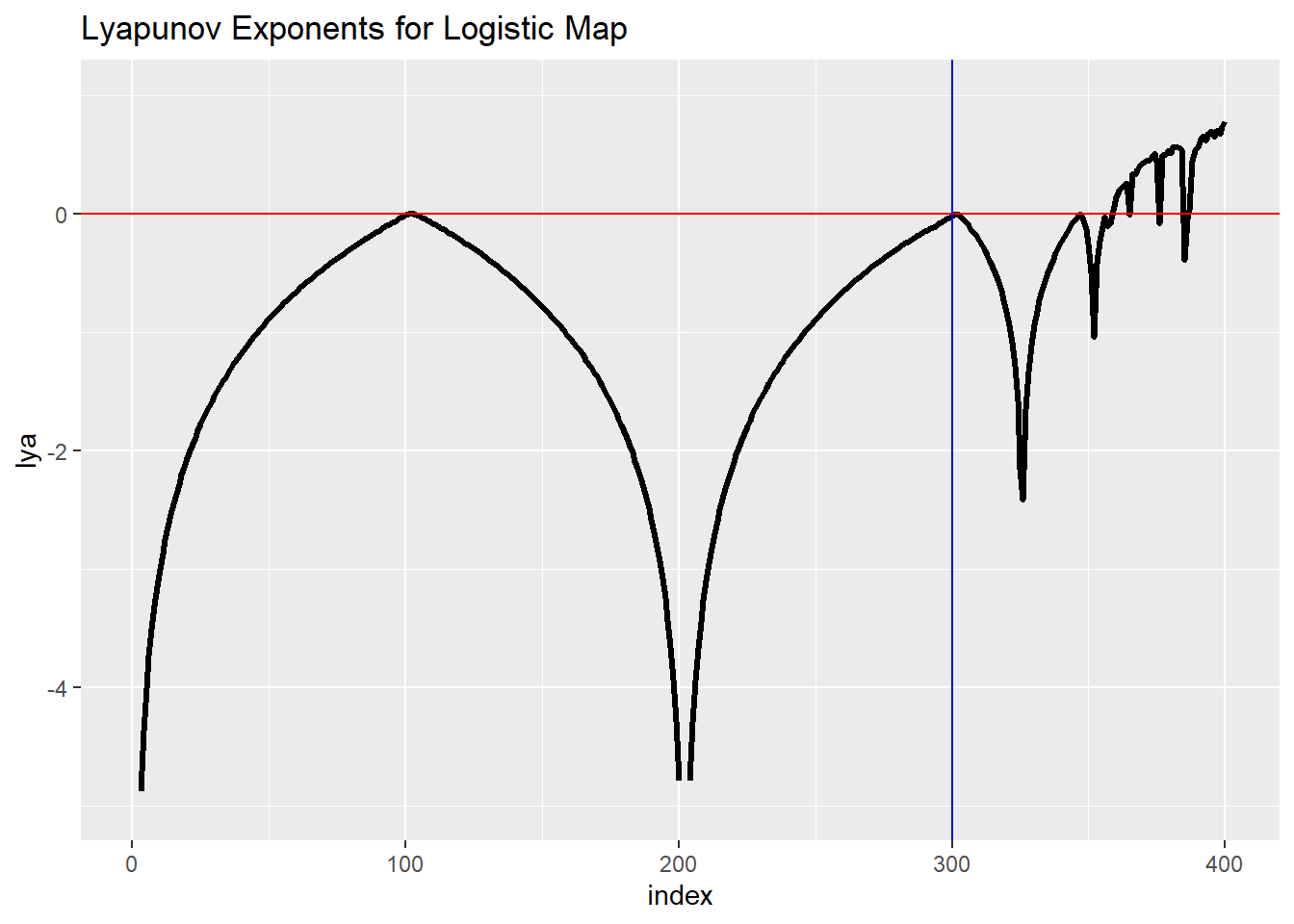# next 3 lines from http://www.magesblog.com/2012/03/logistic-map-feigenbaum-diagram.html:
my.r <- seq(0, 4, by=0.003)
r <- sort(rep(my.r, 301))   # expand my.r since each run of logistic.map generates 300 points
Orbit <- sapply(my.r, logistic.map, x=0.1, N=1000, M=300)
N <- unlist(lapply(seq_len(ncol(Orbit)), function(i) Orbit[,i]))

my_df <- data.frame(r=r, N=N)
ggplot(my_df, aes(x=r, y=N)) + geom_point(size=0.5)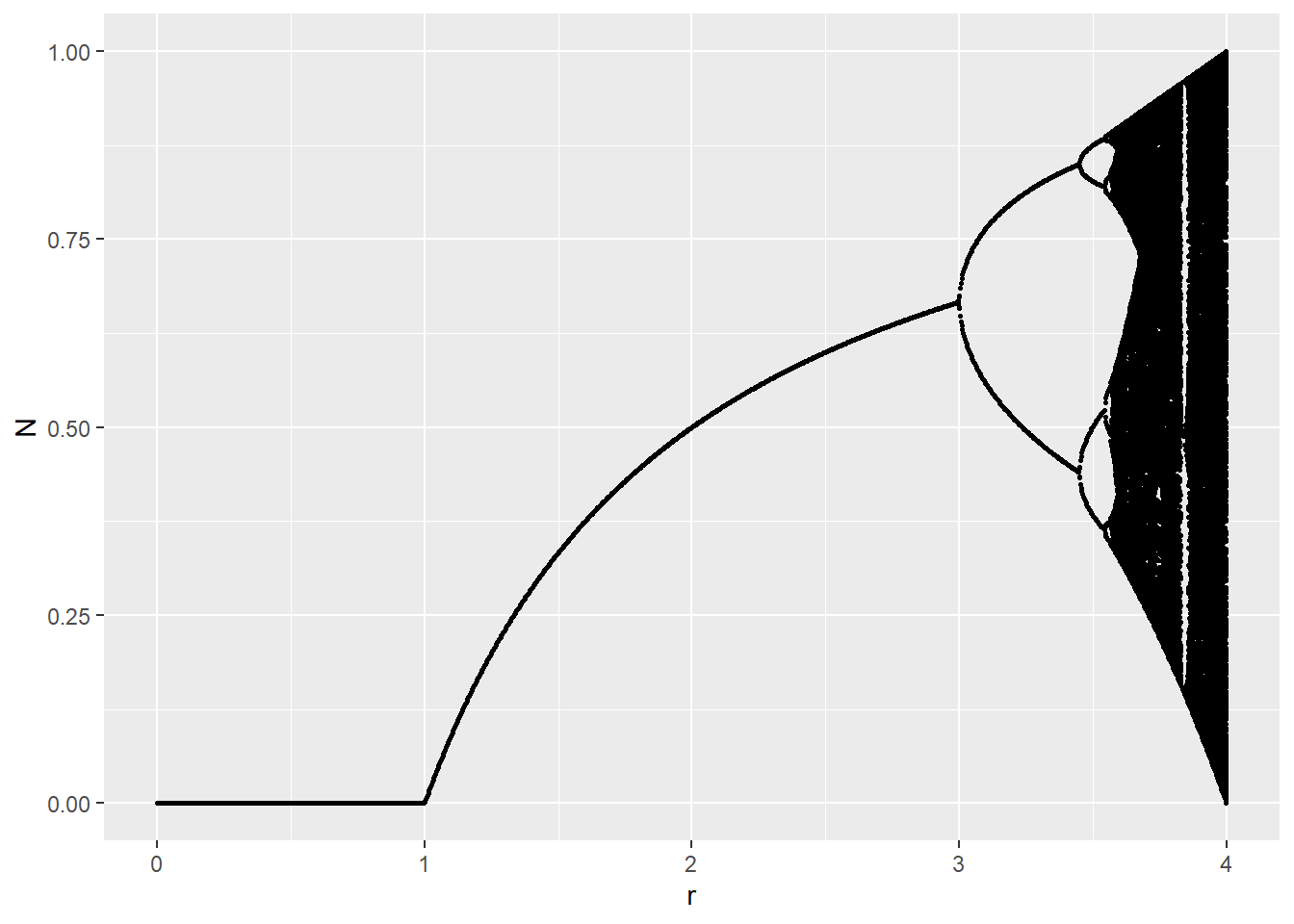# Alternative in Base R:
plot(r, Orbit, pch=".", cex=0.5, main="Bifurcation Diagram for r=0 to r=4 Logistic Maps")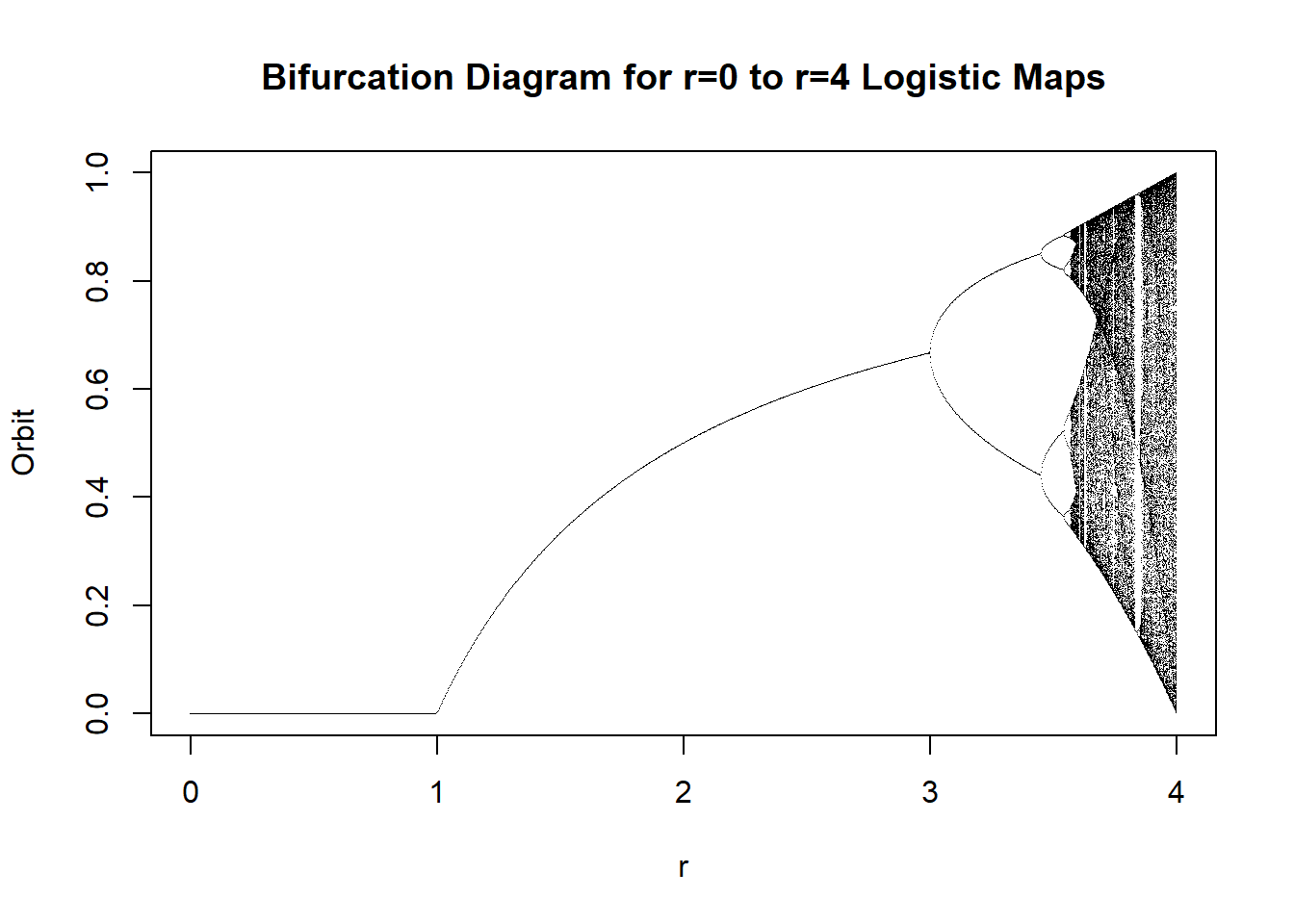## Step 10

Notice that in the bifurcation diagram, we can easily see that when r is between 0 and 1, the population converges to extinction (a population fraction of 0.00). This makes sense, because the growth rate is smaller than what’s required to sustain the size of the population. You might like to zoom in, though, and see what the orbits look like for some smaller portions of the diagram:

ggplot(my_df, aes(x=r, y=N)) + geom_point(size=0.5) + scale_x_continuous(limits=c(3.5,4.0))